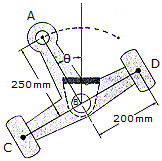# Engineering Mechanics - Planar Kinematics of a Rigid Body (PKRB) - Discussion

### Discussion :: Planar Kinematics of a Rigid Body (PKRB) - General Questions (Q.No.14)

14.Arm ABCD is printed at B and undergoes reciprocating motion such that= (0.3 sin 4t) rad, where t is measured in seconds and the argument for the sine is in radiaus. Determine the largest speed of point A during the motion and the magnitude of the acceleration of point D at this instant.

 [A]. vAmax = 0.0600 m/s, aD = 1.002 m/s2 [B]. vAmax = 0.300 m/s, aD = 0.960 m/s2 [C]. vAmax = 0.0600 m/s, aD = 0.916 m/s2 [D]. vAmax = 0.300 m/s, aD = 0.288 m/s2

Explanation:

No answer description available for this question.